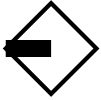﻿ MathJax TeX Test PageLogics for Qualitative Reasoning   Logics for space   Compass logic

## Compass Logic   (written by Przemysław Wałęga)

Formalism description:

Formulas $\varphi$ of compass logic are generated by the following abstract syntax:

$\varphi ::= p \mid \top \mid \neg \varphi \mid \varphi \land \varphi \mid$$\varphi \mid$$\varphi \mid$$\varphi \mid$$\varphi ,$

where $p$ denotes a propositional letter.

Kripke-style semantics:

A model of compass logic is a tuple of the form $\mathfrak{M} = ( T_1 \times T_2, <_1, <_2, V )$, where $T_1, T_2$ are nonempty sets, $(T_1,<_1)$ and $(T_2,<_2)$ are linear orders, and $V: T_1 \times T_2 \to 2^{Var}$ is a valuation that assigns a subset of a set of all propositional variables $Var$ to each point from $T_1 \times T_2$ .

Let $t,s \in T_1$ and $u,v \in T_2$. The satisfaction relation $\models$ in a compass logic model $\mathfrak{M}$ in a point $(t,u)$ is defined as follows:

 $\mathfrak{M},t,u \models \top$ for any $t\in T_1, u \in T_2 ;$ $\mathfrak{M},t,u \models p$ iff $p \in V(t,u);$ $\mathfrak{M},t,u \models \neg \varphi$ iff $\mathfrak{M},t,u \not\models \varphi;$ $\mathfrak{M},t,u \models \varphi \land \psi$ iff $\mathfrak{M},t,u \models \varphi$ and $\mathfrak{M},t,u \models \psi;$ $\mathfrak{M},t,u \models$$\varphi$ iff for some $s \in T_1$ such that $t<_1 s$ we have $\mathfrak{M},s,u \models \varphi;$ $\mathfrak{M},t,u \models$$\varphi$ iff for some $s \in T_1$ such that $s<_1 t$ we have $\mathfrak{M},s,u \models \varphi;$ $\mathfrak{M},t,u \models$$\varphi$ iff for some $s \in T_1$ such that $u<_1 v$ we have $\mathfrak{M},s,u \models \varphi;$ $\mathfrak{M},t,u \models$$\varphi$ iff for some $s \in T_1$ such that $v<_1 u$ we have $\mathfrak{M},s,u \models \varphi;$
Application fields:

Spatial reasoning, interval temporal reasoning.

Compass logic is introduced in (Venema 1990). Its finite axiom system is presented in (Marx & Venema 1997) and a proof of undecidability in (Marx & Reynolds 1999). Compass logic is related to interval logics, namely a point $(x,y)$ in a northwestern halfplane of $T_1 \times T_2$ may be treated as an interval starting in $x$ and ending in $y$. As a result, all modal operators from the interval temporal logic of Halpern-Shoham may be expressed in compass logic (Venema 1990).